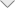### Four-way Microstrip Wilkinson Power Divider Modeling

Introduction

This document demonstrates modeling of Printed Circuit Boards (PCB) with Green Function approach using new powerful tools of EMCoS PCB VLab and EMC Studio. In conjunction, these two products make it easy to build a full simulation chain for various types of PCB models. In this application note Four-way Microstrip Wilkinson Power Divider modeling is considered. Simulated characteristics of structure are compared with reference measurement data.

Simulation Workflow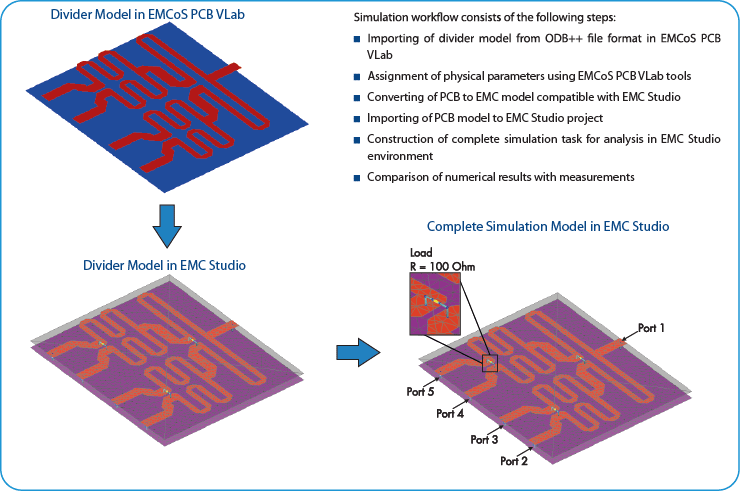Measurement Setup

Measurement Setup is represented by a four-way microstrip Wilkinson power divider on a 2 mm-thick substrate with relative dielectric constant 4.4. The divider uses 3 Wilkinson power divider to go from one input (out) to four outputs (inputs). Parameters of components of setup are listed in table below.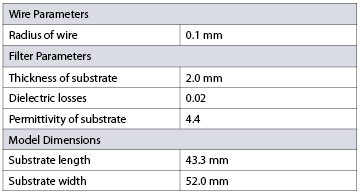Measurements were performed in EMCoS laboratory.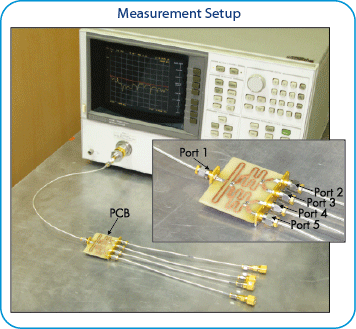Results

The comparison of simulation and measurement results is shown below. There is good agreement between measurement and simulation results in considered frequency range.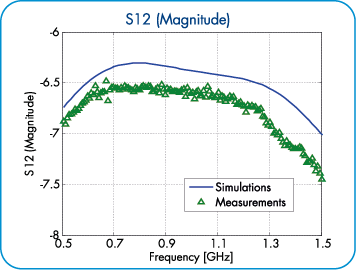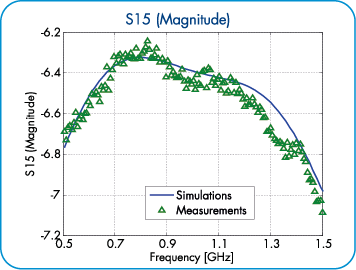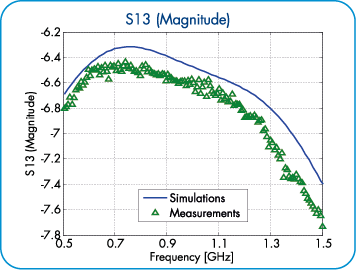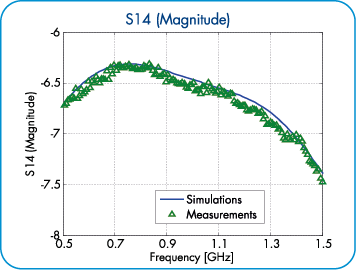The current distribution on Microstrip Wilkinson Power Divider for resonant frequencies is shown below.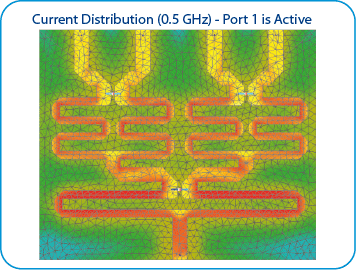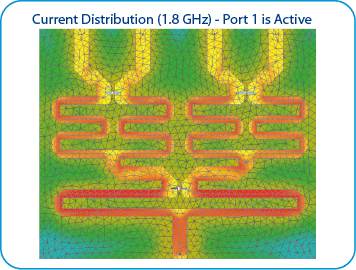Conclusions

According to performed investigation the main conclusions are:

• Computational model of PCB can be effectively generated using EMCoS PCB VLab and EMC Studio
• Simulation results are in good agreement with measurement results
﻿